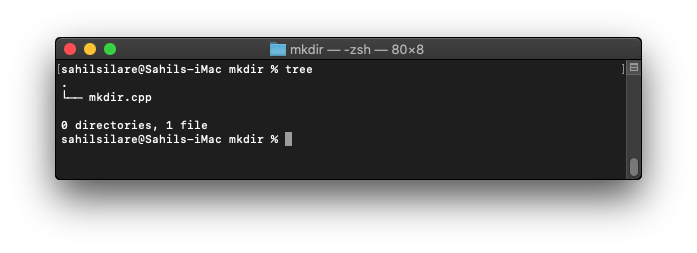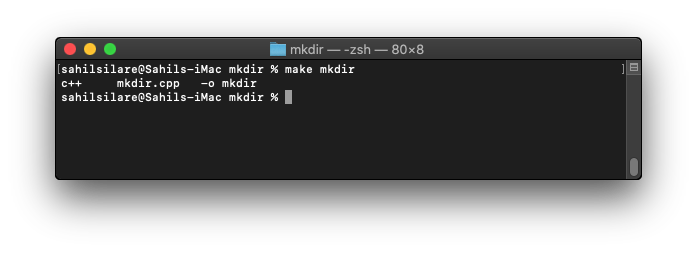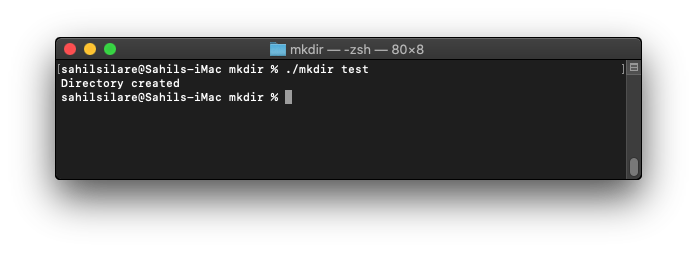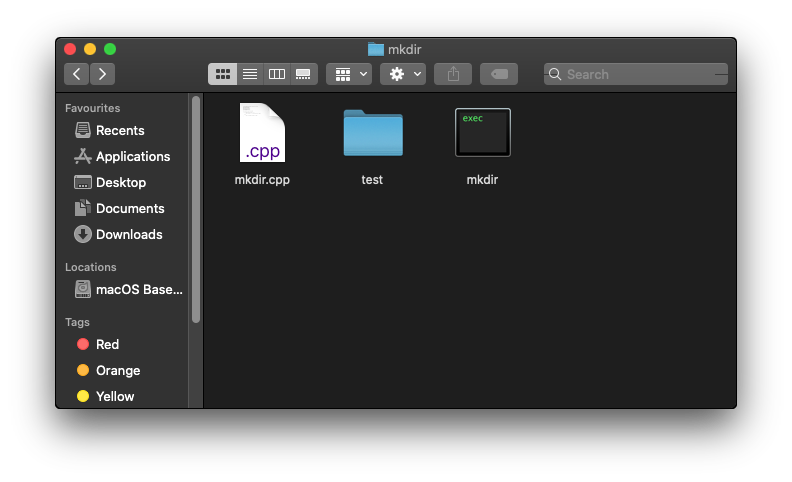Implementing mkdir in C/ C++

Software Engineering C C++Get this book -> Problems on Array: For Interviews and Competitive Programming

Ever thought to make directories without the usual right-click functionality in the operating system?, you can do that by another way which is through command line! The "mkdir" command is exactly made for that!. The "mkdir" command stands for "make directory/ies" . This is an inbuilt command in the UNIX based filesystems and is widely used for saving time by following the usual right-click creation method, let's dive into implementation of the "mkdir" command,

Initial setup

The initial setup follows the usual setting up of C++ file, so create a file named "mkdir.cpp" and here we will write our implementation code,

After the correct setup the directory tree will look like this,Implementation

For the implementation of the logic we will need several header files, namely,

• algorithm : For using the algorithms required in the program.
• sys/stat.h : Used by the existing mkdir function
• sys/types.h : Used for error types and existing mkdir function.

Then let's move to the implementation part,

#include <algorithm>
#include <sys/stat.h>
#include <sys/types.h>
using namespace std;

Now we'll require command line arguments for the successful execution of the command. So we'll define the main function with arguments as,

int main(int argc, char **argv){
//Logic implementation
}

What this does is allows the user to pass the names through command line, such as the name of the directory to be created, so at the time of execution we could pass the name as,

\$ ./filename argument1

Here argv signifies the list of arguments passed,

Now we'll use the prebuilt function mkdir in C++ to create a directory, this function takes two arguments, the first one being the filename and the second one is the file permissions for user,group and others.

int main(int argc, char **argv){
//Logic implementation
if(mkdir(argv,0777) == -1)
cerr << "Error : " << strerror(errno) << endl;
}

The permissions 0777 stands for the permission type, 7 stands for the user permissions that the user could read, write, and excute the file. If the file is not present the function will create a directory, else will return -1. The argument argv stands for the directory name that you want to be created here for example we are going to use test as the argv but you could use it as anything you wish for.

Now what if the directory doesn't exist? You guessed it right! We'll create one!

int main(int argc, char **argv)

{
if (mkdir(argv, 0777) == -1)
cerr << "Error : " << strerror(errno) << endl;

else
cout << "Directory created" << endl;
}

So the complete implementation of the file will look like,

// C++ program to create a directory in Linux
#include <algorithm>
#include <sys/stat.h>
#include <sys/types.h>
using namespace std;

int main(int argc, char **argv)

{
if (mkdir(argv, 0777) == -1)
cerr << "Error : " << strerror(errno) << endl;

else
cout << "Directory created";
}

For running the file, we'll use terminal, or you could do it with any compiler of your choice,

Here I'll use terminal and make command to compile the file,Then to run the file we'll use ./mkdir along with the filename,

\$ ./mkdir testNow by listing the filesystem we could see that the directory named test has been created!So here our implementation is concluded and is working successfully in creating the directories!Sahil Silare

B.Tech Student in Computer Science at National Institute of Technology (NIT), Raipur (2019 - 2023) | Intern at OpenGenus